Pair Distribution Function (PDF) Analysis
- Practical Electron Microscopy and Database -
- An Online Book -
Microanalysis | EM Book                                                                                   http://www.globalsino.com/EM/

=================================================================================

In a gas, liquid, or solid, the average number density of atoms can be given by,
ρ0 = n/v -------------------- [3097a]
where,
n -- The total number of atoms.
v -- The total volume.
Then, the number density of atoms at a distance r from an origin atom will be given by ρ0g(r). Here, g(r) is called pair distribution function (PDF), which in fact is a probability density function. PDF is radially averaged and thus is an one-dimensional (1-D) function.

PDF analysis describes a probability of finding any two atoms at given inter-atomic distances r, also called a distance map inside a solid. The probability is then given by ρ0g(r)dr. Figure 3097a shows the schematic illustration of g(r) dependence on r. The function may oscillate about 1 until gradually approaching 1. The magnitude of the oscillations directly reflects the lower or higher density at r.Figure 3097a. Schematic illustration of g(r) dependence on r.

For PDF, each peak is assigned to every atom j at the position corresponding to the interatomic distance rij between atoms i and j. Therefore, we can have PDF given by,--------------------------- [3097a]--------------------------- [3097b]
where,
bi and bj -- The scattering lengths for atom i and j, respectively.
<b> -- The average scattering length over all atoms.
ρ0 -- The number density.
ρ(r) -- The pair density function.
The measured scattering intensity can be given by,--------------------------- [3097c]--------------------------- [3097d]
Then, PDF can be obtained through direct Fourier transform,--------------------------- [3097e]
where,
Q -- The scattering vector.

Similar to radial distribution function (RDF), PDF is also related to the Fourier transform of the measured scattering intensity S(Q), also called structure function, as indicated in Equation 3097e. For the same reason, g(r) is often obtained by Fourier transformation of the total structure factor that is measured in neutron, X-ray and electron scattering experiments, and thus analysis of liquid and amorphous structures is usually based on g(r).

Figure 3097b shows the schematic illustration of pair distribution functions of crystal, amorphous, liquid, and gas states. In principle, the PDF of crystals should ideally be represented by vertical lines due to long-range ordering; however, these lines are broadened because of thermal vibrations of atoms and defects in real crystals. Liquids and amorphous solids are arranged in short-range ordering and the nearest coordination shells are still visible, while the others are destroyed with increasing r due to lack of long-range ordering. For a gas with random positions of the atoms, there is equal probability in the radial distribution for r > d. Here, d is the minimum distance between the pairs of the particles due to repulsive forces.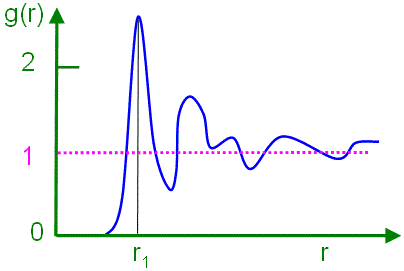(a) (b)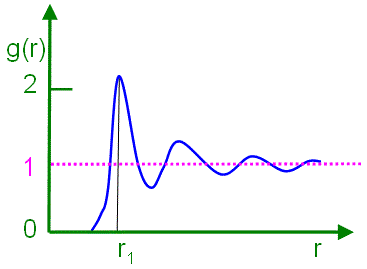(c) (d)
 Figure 3097b. Schematic illustration of pair distribution functions of (a) crystal, (b) amorphous, (c) liquid, and (d) gas states.

Figure 3097c shows the schematic illustrations of generation of pair distribution function from a two-dimensional (2-D) crystal and the corresponding peaks in the radial distribution function.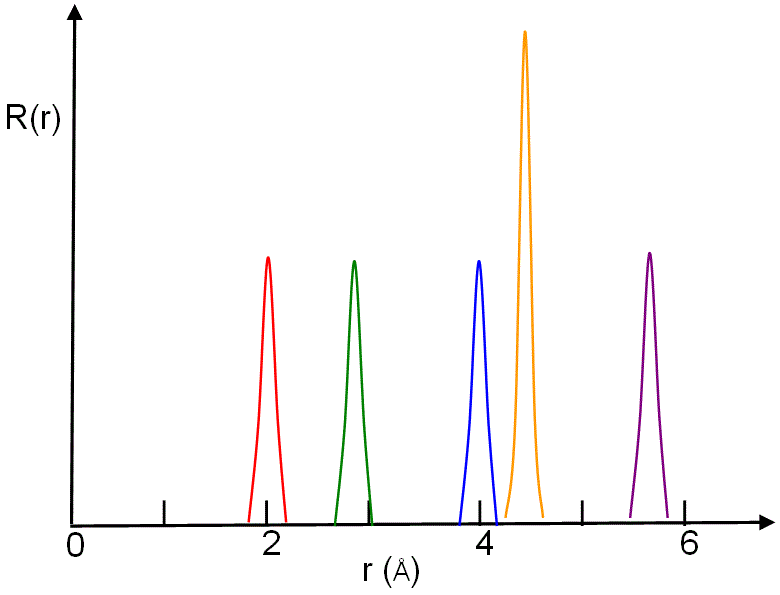(a) (b)
 Figure 3097c. Schematic illustrations of (a) generation of pair distribution function from a two-dimensional (2-D) crystal (the colored circles indicate distances of neighbours to the origin atom), and (b) corresponding peaks in the radial distribution function in the same color.

Figure 3097d shows the pair distribution function g(r), of the fcc gold (Au) crystal, from the black (origin) atom at room temperature. The PDF peaks with atoms in different colors correspond to the atoms in the same color in the atomic structure of the inset.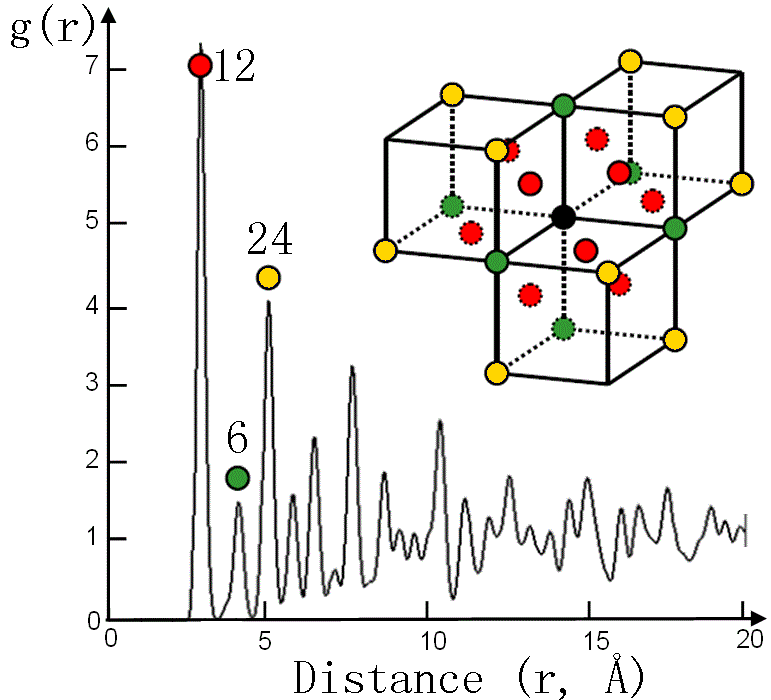Figure 3097d. Pair distribution function g(r) of fcc gold (Au) at room temperature.

Figure 3097e shows the PDF of crystalline nickel (Ni) simulated with molecular dynamics (MD) model for two different temperatures. In general, the main peaks at higher temperatures shifts to higher r.Figure 3097e. PDF of crystalline Ni simulated with molecular dynamics model. Adapted from 

Figure 3097f shows comparison between the pair distribution functions g(r) for Au and Ni liquids.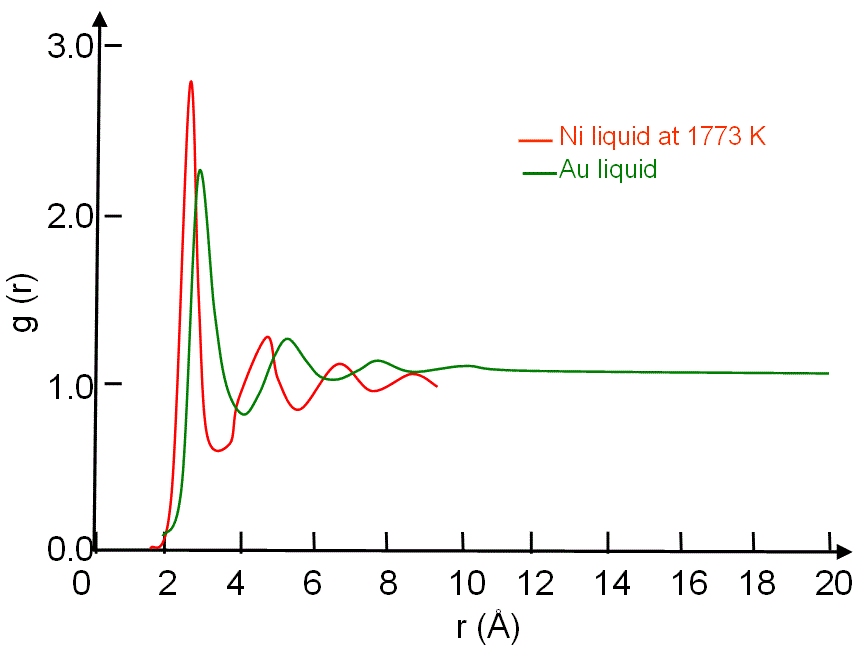Figure 3097f. Comparison between the pair distribution functions g(r) for Au and Ni liquids.

In both ordered (e.g. crystal) and disordered (e.g. amorphous) states, g(r) can be used to evaluate the average number (n) of atoms located between r1 and r2,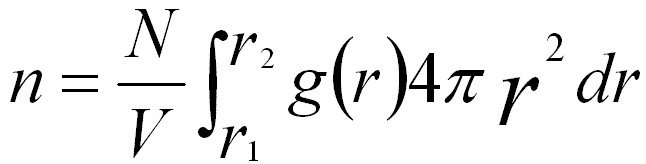---------------------- [3097f]
where,
N -- The total number of atoms.
V -- The total volume of the material.

For instance, Figure 3097d shows the numbers of first (12), second (6) and third (24) nearest atoms in FCC Au crystal.

The pair distribution function, including the full information about the three-dimensional (3-D) structure, is difficult to plot because of the dependence on vectors. Therefore, in practice, this information is reduced to the radial distribution function. In this case, the PDF can be determined by synchrotron X-ray or neutron diffraction data. PDF analysis is often used to study SRO (short range ordering) in amorphous or crystalline materials as well as liquids. For crystals, PDF is a powerful technique to determine the deviation of SRO from the average crystal structure. Table 3097 summarizes the comparison between PDF method and powder X-ray diffraction.

Table 3097. Comparison between PDF method and powder X-ray diffraction.

 PDF method Powder X-ray diffraction Analyzed signals Both Bragg peaks (from long-range ordering) and diffuse scattering (from local/temporal deviation from the long-range ordered structure) Bragg peaks (from long-range ordering) only Applications Nano-crystalline and noncrystalline materials Mainly crystalline materials

 J. Lu and J. A. Szpunar, Phil. Mag. A 75, 1057-1066 (1997).

=================================================================================